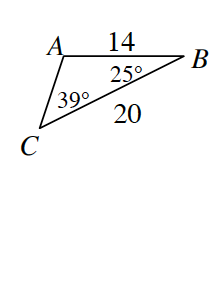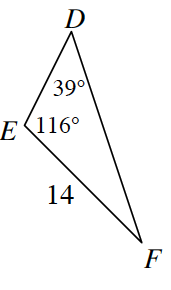### Home > INT1 > Chapter 7 > Lesson 7.1.3 > Problem7-36

7-36.

Examine $ΔABC$ and $ΔDEF$ at right.

1. Complete a flowchart to justify the relationship between the two triangles. Assume the triangles at right are not drawn to scale.

Use the Triangle Angle Sum Theorem to find the unknown angle in each triangle.
2. Determine ${DF}$.